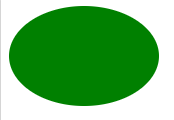Open in App
Not now

# How to draw an ellipse using CSS ?

• Last Updated : 31 May, 2021

In this article, we will learn how to create an ellipse using CSS. To create an ellipse first we will create a simple rectangle of our desired height and width.

Approach To create Rectangle: In order to create an ellipse of our desired size, we will create a div in HTML and will give it a class named as an ellipse. Now we will set the height and width of the div, it will look like a rectangle. We can set the background color as per our choice.

Below is the implementation to create the rectangle.

## HTML

 ```<``html``>``   ` `<``head``>``    ````    ``<``style``>`` ` `        ``/* Setting Height and Width of Rectangle */``        ``.ellipse{``            ``height: 100px;``            ``width: 150px;``            ``background-color: green;``        ``}``    `````` ` `<``body``>`` ` `    ````    ``<``div` `class``=``"ellipse"``>```` ` ``

Output:Rectangle

Approach to Create Ellipse from Rectangle: Now to convert this rectangle into an ellipse, we will set the border-radius refers to a very high value (50% to 100%). It refers to the curvature at the corners of the ​shape. The height of the rectangle will be the minor axis of the ellipse and the width will be the major axis.

## HTML

 ```<``html``>``   ` `<``head``>`` ` `    ````    ``<``style``>`` ` `        ``/* Setting Height and Width of Rectangle */``        ``.ellipse{``            ``height: 100px;``            ``width: 150px;``            ``background-color: green;`` ` `            ``/* Setting Border Radius */``            ``border-radius: 50%;``        ``}``    `````` ` `<``body``>`` ` `    ````    ``<``div` `class``=``"ellipse"``>```` ` ``

Output:Ellipse

My Personal Notes arrow_drop_up